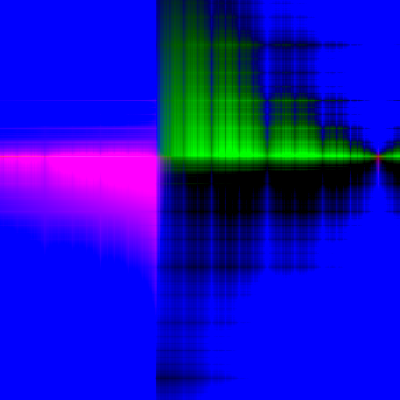# Virtual Brownian Motion and Sheet samplers

This demo implements algorithms for stateless, constant-memory sampling of entire functions from a Brownian motion of any dimension. This kind of sampler is useful in adaptive stochastic partial differential equation solvers, since it allows the noise to be sampled in a stateless manner.

This code also demonstrates Dex's ability to do fast stateful for loops, split random keys in a fine-grained way, and how to use the typeclass system to implement a form of recursion.

## One-dimensional Brownian Motion on the Unit Interval

The function below implements the virtual Brownian tree algorithm from Scalable Gradients for Stochastic Differential Equations. It lazily evaluates the value of a function sampled from a Brownian bridge up to a specified input tolerance.

The rest of the algorithms in this file simply translate and scale calls to this function.

struct SamplerState(v) = k : Key y : v sigma : Float t : Float
def sample_unit_brownian_bridge( tolerance:Float, sampler: (Key)->v, key:Key, t:Float ) -> v given (v|VSpace) = -- Can only handle t between 0 and 1. -- iteratively subdivide to desired tolerance. num_iters = 10 + f_to_n (-log tolerance) init_state = SamplerState(key, zero, 1.0, t) result = fold init_state \i:(Fin num_iters) prev. [key_draw, key_left, key_right] = split_key key -- add scaled noise t' = abs (prev.t - 0.5) new_y = prev.y + prev.sigma * (0.5 - t') .* sampler key_draw -- zoom in left or right new_key = if prev.t > 0.5 then key_left else key_right new_sigma = prev.sigma / sqrt 2.0 new_t = t' * 2.0 SamplerState(new_key, new_y, new_sigma, new_t) result.y

### One-dimensional Brownian motion anywhere on the real line

These functions generalize the sampling of a Brownian bridge on the unit interval to sample from a Brownian motion defined anywhere on the real line. When evaluating at times larger than 1, an iterative doubling procedure eventually produces values bracketing the desired time. These bracketing values are then used to call the iterative Brownian bridge sampler.

def scale_brownian_bridge(unit_bb:(Float)->v, x0:Float, x1:Float, x:Float) -> v given (v|VSpace) = sqrt (x1 - x0) .* unit_bb ((x - x0) / (x1 - x0))
def linear_interp(t0:Float, x0:v, t1:Float, x1:v, t:Float) -> v given (v|VSpace) = unit_t = (t - t0) / (t1 - t0) (1.0 - unit_t) .* x0 + unit_t .* x1
def sample_brownian_bridge( tolerance:Float, sampler: (Key)->v, key:Key, t0:Float, y0:v, t1:Float, y1:v, t:Float ) -> v given (v|VSpace) = -- Linearly interpolate between the two bracketing samples -- and add appropriately scaled Brownian bridge noise. unit_bb = \t. sample_unit_brownian_bridge (tolerance / (t1 - t0)) sampler key t bridge_val = scale_brownian_bridge unit_bb t0 t1 t interp_val = linear_interp t0 y0 t1 y1 t bridge_val + interp_val
def sample_brownian_motion( tolerance:Float, sampler: (Key)->v, key:Key, t':Float ) -> v given (v|VSpace) = -- Can handle a t' anywhere on the real line. -- Handle negative times by reflecting and using a different key. [neg_key', key'] = split_key key (key, t) = if t' < 0.0 then (neg_key', -t') else (key', t') [key_start, key_rest] = split_key key first_f = sampler key_start -- Iteratively sample a point twice as far ahead -- until we bracket the query time. doublings = Fin (1 + (natlog2 (f_to_n t))) init_state = (0.0, zero, 1.0, first_f, key_rest) (t0, left_f, t1, right_f, key_draw) = fold init_state \i:doublings state. (t0, left_f, t1, right_f, key_inner) = state [key_current, key_continue] = split_key key_rest new_right_f = left_f + (sqrt t1) .* sampler key_current (t1, right_f, t1 * 2.0, new_right_f, key_continue) -- The above iterative loop could be mostly parallelized, but would -- require some care to get the random keys right. sample_brownian_bridge tolerance sampler key_draw t0 left_f t1 right_f t

## Demo: One-dimensional Brownian motion

import plot
pixels = Fin 400
xs = linspace pixels 0.3 2.1
tolerance = xs[1@_] - xs[0@_]
fs = each xs \x. sample_brownian_motion tolerance randn (new_key 0) x

### Generalize the output type to any dimension

This typeclass lets the output type of the Brownian sheet be anything for which a Gaussian sampler can be written.

interface HasStandardNormal(a:Type) rand_normal : (Key) -> a
instance HasStandardNormal(Float32) def rand_normal(k) = randn k
instance HasStandardNormal(n=>a) given (a|HasStandardNormal, n|Ix) def rand_normal(k) = for i. rand_normal (ixkey k i)

### Generalize the input type to any dimension

Suprisinly, a sampler for 1D Brownian motion can easily be extended to sample from a Brownian sheet of any dimension. We simply have to replace the sampler used for scalar-valued standard Gaussian noise with one that samples entire functions from a Gaussian process. In particular, we can re-use the 1D Brownian bridge sampler that we wrote above. This process can be nested to arbitrarily many dimensions.

interface HasBrownianSheet(a:Type, v|HasStandardNormal) -- tolerance -> key -> input -> output sample_brownian_sheet : (Float, Key, a) -> v
instance HasBrownianSheet(Float, v) given (v|HasStandardNormal|VSpace) def sample_brownian_sheet(tol, k, x) = sample_brownian_motion tol rand_normal k x
instance HasBrownianSheet((a, Float), v) given (a, v|VSpace) (HasBrownianSheet a v) def sample_brownian_sheet(tol, k, xab) = -- the call to sample_brownian_sheet below will recurse -- until the input is one-dimensional. (xa, xb) = xab sample_a = \k. sample_brownian_sheet tol k xa sample_brownian_motion tol sample_a k xb

## Demo: Two-dimensional Brownian sheet

Next, we plot a single sample from a two-dimensional Brownian sheet with a 3-dimensional (red, green, blue) output.

import png
samp : pixels=>pixels=>(Fin 3)=>Float = for i j. sample_brownian_sheet tolerance (new_key 0) (xs[i], xs[j])
:html imshow samp